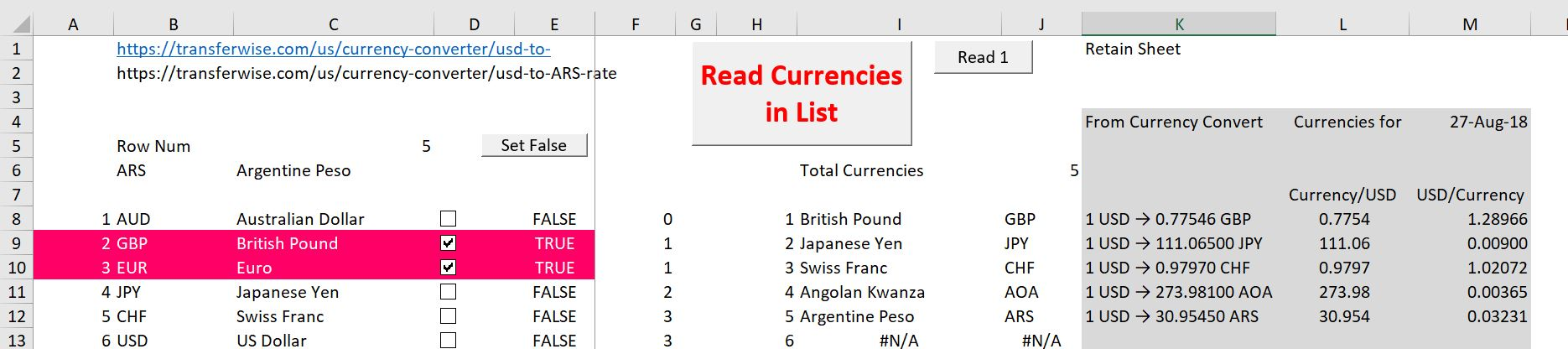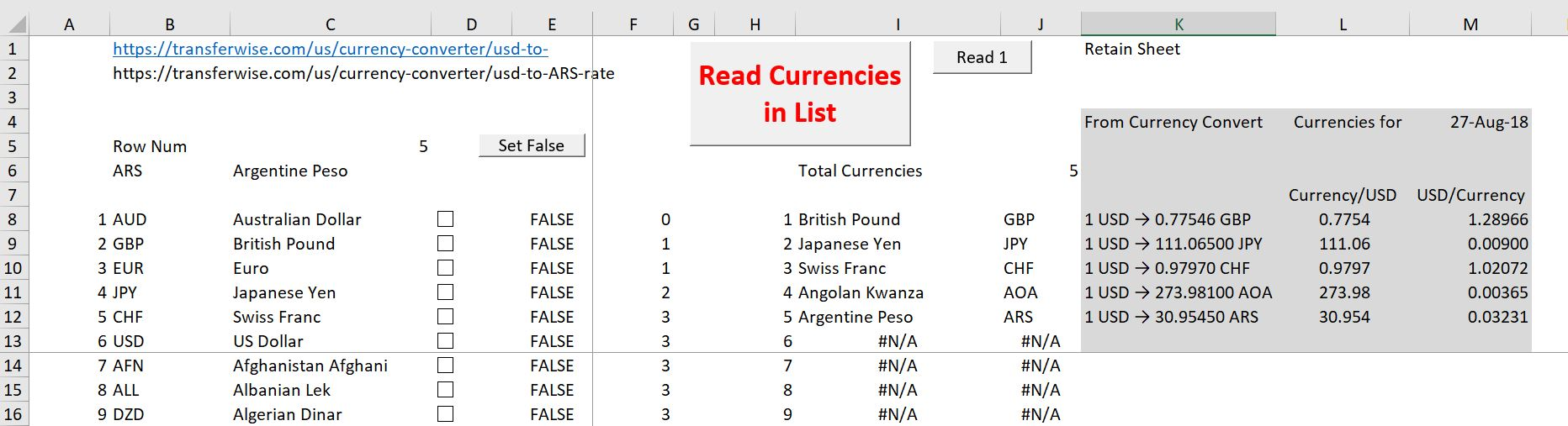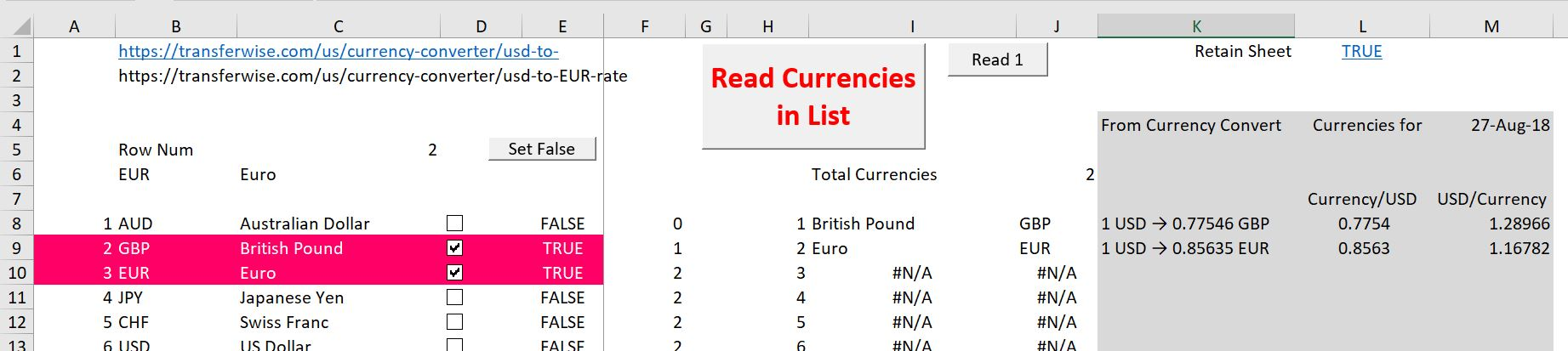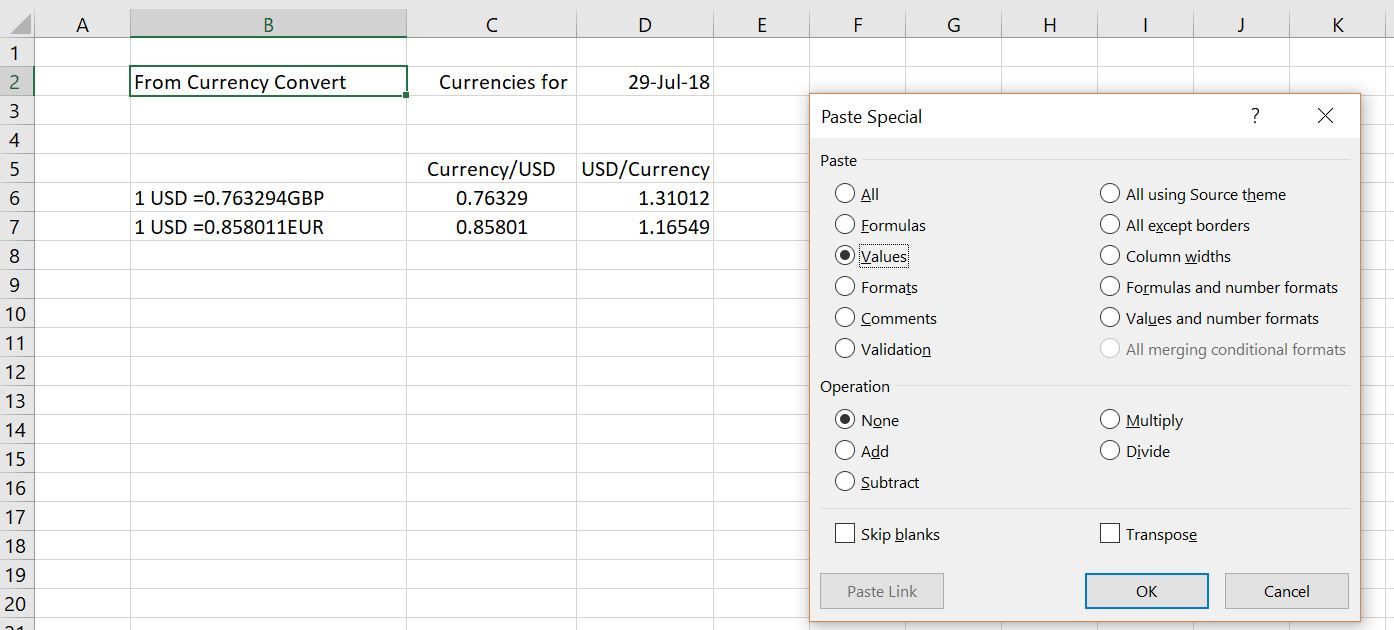# Using Current Currency Database For Invoices etc.

This sheet demonstrates how you can use, modify and create a file that downloads selected currencies.  After downloading the file you can quickly extract different currencies and copy the data into an excel file after clicking a couple of check boxes.  The file uses the workbooks.open method as well as multiple check boxes.

## Introduction

The file that you can download below uses current exchange rates from https://transferwise.com/us/currency-converter/usd-to-EUR-rate.  It formerly used the website http://www.xe.com/currencyconverter/convert/?From=USD&To=CHF but this website no longer allows you to scrape anything.

In the completed file below, you can select different exchange rates and press a button (Read Currencies in List) to get current updates of the exchange rate.  I have used the technique to use check boxes with TRUE and FALSE and Match as well as a macro that turns off all of the TRUE’s in all of the checkboxes.

If you download the file below, all you have to do is click on the currencies you want with the check boxes. Then click the macro box and all of your exchange rates are ready to be copied into any other file that you want.

## Instructions for Using the Currency File

When you open the file, you may see something like what is in the screenshot below.  This screen shot has some existing data from a prior run.  I had begun clicking some currencies that I was interested in downloading.  Note the final currencies are included in the grey box with the date that you have downloaded the data.  This data in the grey box can be copied and pasted as values to your file.The second screenshot shows how all the checkboxes are unclicked after pressing the button name “Set to False.”  You can see that all of the boxes are blanks.  In this case no currencies are selected.The third screenshot shows how after selecting Sterling and Euro, you get a new summary.  You just press the button named “Read Currencies in List”.  Technical details that explain how to create the macro to scrape the data that are part of the macro assigned to this button are shown in the next section.## Technical VBA Details

In this section I have copied the VBA code that uses the workbooks.open technique to get the data.  The file works like other files in that you separate and make new URL’s and use the INDEX function.  Once you have the URL, the loop works through each currency, reads the currency from the internet.  The row for 1 USD is found and the entire row is copied to the base file.

.

.

```Public row_output_number As Single
Public currency_number As Single
Public base_sheet As String

Sub all_currencies()

Application.ScreenUpdating = False ' dont show the screens

current_calc = Application.Calculation

Application.Calculation = xlCalculationSemiautomatic

For Sheet = Sheets.Count To 1 Step -1

If Sheets(Sheet).Name = "Break Sheet" Then
Exit For
End If

Worksheets(Sheet).Delete

Next Sheet

Application.Calculation = xlCalculationSemiautomatic

base_sheet = ActiveSheet.Name

date_output = DateValue(Now) ' if you want to show the date somewhere

Range("date_out") = date_output ' put the title date at to
Range("date_out").Select
Selection.NumberFormat = "d-mmm-yy"
Range("title_1") = "Currencies for "

row_output_number = 200

Range("output").Clear

Range("total_currencies").Calculate

MsgBox " This puts out beginning in row 200 " & Chr(13) & Chr(13) & " Reading " & Range("total_currencies") & " currencies"

For i = 1 To Range("total_currencies") ' from counta

Range("row_num") = i

row_output_number = row_output_number + 1

Next i

Application.StatusBar = False ' put the url name at the bottom

Sheets(base_sheet).Select
Application.Calculation = current_calc

End Sub

Dim test_value, test_criteria As String

close_file = Range("close_file") ' Read option from sheet

current_book = ActiveWorkbook.Name ' record the existing location
current_sheet = ActiveSheet.Name

Application.StatusBar = Range("url_name") ' put the url name at the bottom

url_name = Range("url_name") ' each url name in the range name

Workbooks.Open (url_name) ' read the file

temp_book_name = ActiveWorkbook.Name ' define the current sheet for closing later on

Cells.Select ' copy the sheet without ads etc
Selection.Copy
Selection.PasteSpecial Paste:=xlPasteValues, Operation:=xlNone, SkipBlanks _
:=False, Transpose:=False

test_value = "1 USD" ' What to Look For
test_criteria = "1 USD" ' What to Look For

evaluation = StrComp(test_value, "1 USD", vbTextCompare) ' evaluation is zero when equal

evaluation = StrComp(test_value, test_criteria, vbTextCompare) ' evaluation is zero when equal

For Row = 1 To 150 ' look for the exchange rate

test_value = Range("A:A").Cells(Row, 1) ' Look in Column A
test_value = Left(test_value, 5)
evaluation = StrComp(test_value, test_criteria, vbTextCompare)

If evaluation = 0 Then ' Zero is when found in the STRCOMP Function
match_truncated = Row ' Retain the Row Number
Exit For
End If
Next Row

row_number = Row ' find while in temp sheet

row_name = row_number & ":" & row_number ' row name for copying

Range(row_name).Select ' Select entire row
Selection.Copy ' Copy the entire row

If row_output_number = 0 Then row_output_number = 200

target_cell = "A" & row_output_number ' where to place the result
target_cell = row_output_number & ":" & row_output_number ' where to place the result

Sheets(base_sheet).Activate
Range(target_cell).Select

'
' Paste the single row from the currency URL
'
Sheets(base_sheet).Select

Selection.PasteSpecial Paste:=xlPasteValues, Operation:=xlNone, SkipBlanks _
:=False, Transpose:=False

If close_file Then
Workbooks(temp_book_name).Close ' close the file from the URL
Else
Workbooks(temp_book_name).Activate

Cells.Select ' copy the sheet without ads etc
Selection.Copy

Selection.PasteSpecial Paste:=xlPasteValues, Operation:=xlNone, SkipBlanks _
:=False, Transpose:=False

On Error Resume Next
ActiveSheet.Name = Range("currency")

Workbooks(temp_book_name).Close ' close the file from the URL

ActiveSheet.Move After:=Sheets(Sheets.Count)

End If

Range(current_cell).Select

End Sub

Sub ListBox2_Change()

For i = 1 To 10 ' decide how many possible items on the graph
Range("list_out1").Cells(i, 1).Clear ' clear out the range name that will be re-filled
Next i ' note this output is named list_out2; it can be renamed

ActiveSheet.Shapes.Range(Array("List Box 2")).Select ' change this name to the number of your box

Row = 1 ' Make a row counter to put output

For i = 1 To Selection.ListCount ' This is the number in the list box
If Selection.Selected(i) = True Then ' Only keep the selected items

Range("list_out1").Cells(Row, 1) = Selection.List(i)

Row = Row + 1 ' Assign to the range name
End If
Next i ' Finish loop around the listbox items

Range(base_cell).Select
Range(base_cell).Activate

End Sub

```

## Video Explanation of the Macro and Using the File

The video below shows you how to create a macro that finds the correct place in the file that is downloaded from the internet with the workbooks.open statement and how to set-up the file to download multiple exchange rates.